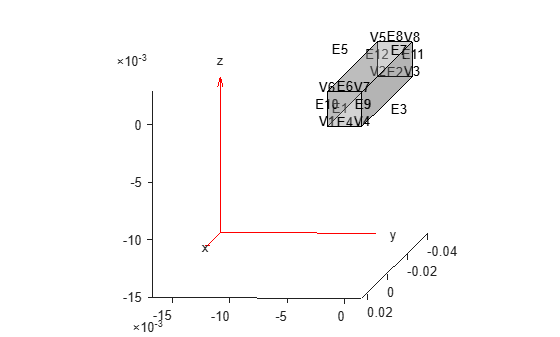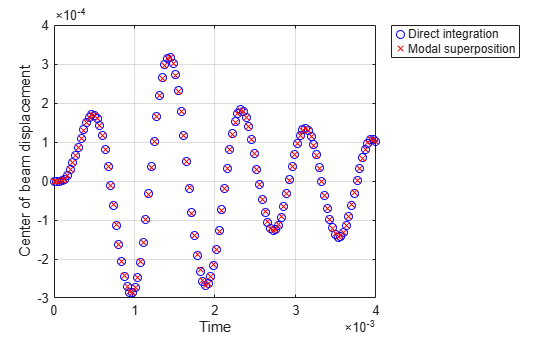# Modal Superposition Method for Structural Dynamics Problem

This example shows how to solve a structural dynamics problem by using modal analysis results. Solve for the transient response at the center of a 3-D beam under a harmonic load on one of its corners. Compare the direct integration results with the results obtained by modal superposition.

### Modal Analysis

Create a modal analysis model for a 3-D problem.

`modelM = createpde('structural','modal-solid');`

Create the geometry and include it in the model. Plot the geometry and display the edge and vertex labels.

```gm = multicuboid(0.05,0.003,0.003); modelM.Geometry=gm; pdegplot(modelM,'EdgeLabels','on','VertexLabels','on'); view([95 5])```Generate a mesh.

`msh = generateMesh(modelM);`

Specify the Young's modulus, Poisson's ratio, and mass density of the material.

```structuralProperties(modelM,'YoungsModulus',210E9, ... 'PoissonsRatio',0.3, ... 'MassDensity',7800);```

Specify minimal constraints on one end of the beam to prevent rigid body modes. For example, specify that edge 4 and vertex 7 are fixed boundaries.

```structuralBC(modelM,'Edge',4,'Constraint','fixed'); structuralBC(modelM,'Vertex',7,'Constraint','fixed');```

Solve the problem for the frequency range from 0 to 500000. The recommended approach is to use a value that is slightly smaller than the expected lowest frequency. Thus, use -0.1 instead of 0.

`Rm = solve(modelM,'FrequencyRange',[-0.1,500000]);`

### Transient Analysis

Create a transient analysis model for a 3-D problem.

`modelD = createpde('structural','transient-solid');`

Use the same geometry and mesh as for the modal analysis.

```modelD.Geometry = gm; modelD.Mesh = msh;```

Specify the same values for the Young's modulus, Poisson's ratio, and mass density of the material.

```structuralProperties(modelD,'YoungsModulus',210E9, ... 'PoissonsRatio',0.3, ... 'MassDensity',7800);```

Specify the same minimal constraints on one end of the beam to prevent rigid body modes.

```structuralBC(modelD,'Edge',4,'Constraint','fixed'); structuralBC(modelD,'Vertex',7,'Constraint','fixed');```

Apply a sinusoidal force on the corner opposite the constrained edge and vertex.

```structuralBoundaryLoad(modelD,'Vertex',5, ... 'Force',[0,0,10], ... 'Frequency',7600);```

Specify the zero initial displacement and velocity.

`structuralIC(modelD,'Velocity',[0;0;0],'Displacement',[0;0;0]);`

Specify the relative and absolute tolerances for the solver.

```modelD.SolverOptions.RelativeTolerance = 1E-5; modelD.SolverOptions.AbsoluteTolerance = 1E-9;```

Solve the model using the default direct integration method.

```tlist = linspace(0,0.004,120); Rd = solve(modelD,tlist)```
```Rd = TransientStructuralResults with properties: Displacement: [1x1 FEStruct] Velocity: [1x1 FEStruct] Acceleration: [1x1 FEStruct] SolutionTimes: [0 3.3613e-05 6.7227e-05 1.0084e-04 1.3445e-04 ... ] Mesh: [1x1 FEMesh] ```

Now, solve the model using the modal results.

```tlist = linspace(0,0.004,120); Rdm = solve(modelD,tlist,'ModalResults',Rm)```
```Rdm = TransientStructuralResults with properties: Displacement: [1x1 FEStruct] Velocity: [1x1 FEStruct] Acceleration: [1x1 FEStruct] SolutionTimes: [0 3.3613e-05 6.7227e-05 1.0084e-04 1.3445e-04 ... ] Mesh: [1x1 FEMesh] ```

Interpolate the displacement at the center of the beam.

```intrpUd = interpolateDisplacement(Rd,0,0,0.0015); intrpUdm = interpolateDisplacement(Rdm,0,0,0.0015);```

Compare the direct integration results with the results obtained by modal superposition.

```plot(Rd.SolutionTimes,intrpUd.uz,'bo') hold on plot(Rdm.SolutionTimes,intrpUdm.uz,'rx') grid on legend('Direct integration', 'Modal superposition') xlabel('Time'); ylabel('Center of beam displacement')```## SupportGet trial now